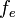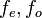# Even part

## Definition

Suppose$f$ is a function whose domain is a subset of the reals that is symmetric about 0, i.e., for every$x$ in the domain of$f$,$-x$ is also in the domain of$f$. Then, the even part of$f$, sometimes denoted$f_e$ or$f_{\operatorname{even}}$ is defined as a function with the same domain, and with the definition:$\! f_e(x) := \frac{f(x) + f(-x)}{2}$

Equivalently, it is the only possible choice of even function in a decomposition of$f$ of the form:$\! f(x) = f_e(x) + f_o(x)$

with$f_e, f_o$ both having the same domain as$f$, and with$f_e$ an even function and$f_o$ an odd function. The other part,$f_o$, is the odd part of$f$.

## Particular cases

Function Domain Even part
polynomial all of$\R$ the sum of the monomials of even degree in that polynomial
exponential function$e^x$ all of$\R$ hyperbolic cosine function$\cosh$